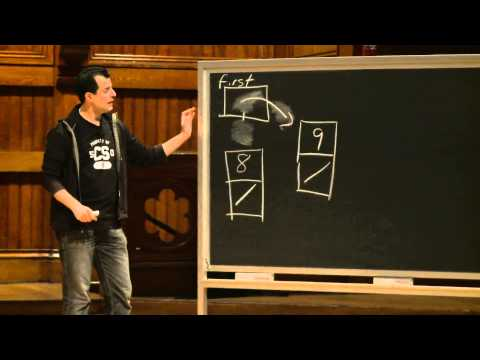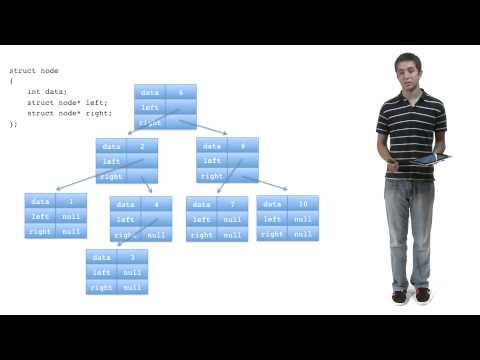# Trees

## Notes

A tree is a data structure in which each node is comprised of some data as well as node pointers to child nodes.

We'll refer to node 1 as the root node, and external nodes like 5, 6, 7, 8, and 9 as leaves.

It's also convention to use the following terms to describe relationships between nodes:

• nodes 2 and 3 are siblings of each other
• nodes 5, 6, and 7 are children of node 3
• node 2 is the parent of node 4

A binary tree is a type of tree in which each node is comprised of some data as well as node pointers to at most two children.

Here's an example of a binary tree node implementation.

Note how each node in a binary tree is comprised of two node pointers and some data.

n is the data stored in this node, and left and right are pointers either to the node's children (or to NULL if no children exist).

A binary search tree is a special type of binary tree that simplifies searching.

For each node in a binary search tree, every value on its left child's side is less than its own value, and every value on its right is greater. Notice how all values to the left of the root (55) are less than 55, and all the values to the right are greater than 55.

Here's some pseudocode to search a binary search tree for a particular value val.

If the current node's value is less than the value you're looking for, recursively search its right child. If the current node's value is greater than the value you're looking for, recursively search its left child.## Slides ( / )## Binary Tree Insertion

Prerequisites:

Implement a function with prototype

``bool insert(int val)``

that inserts a node containing `val` into the tree pointed to by the global `root` variable. Return `true` if successful, and return `false` if you failed for some reason (e.g., lack of sufficient heap memory, value already in tree, etc.).

``````jharvard@run.cs50.net (~): ../a.out
Inserting 0 ...success!
Inserting 1 ...success!
Inserting 2 ...success!
Inserting 3 ...fail!
Inserting 4 ...success!
Inserting 5 ...success!
Inserting 6 ...fail!
Inserting 7 ...fail!
Inserting 8 ...success!
Inserting 9 ...fail!
Tree contains 0? true
Tree contains 1? true
Tree contains 2? true
Tree contains 3? true
Tree contains 4? true
Tree contains 5? true
Tree contains 6? true
Tree contains 7? true
Tree contains 8? true
Tree contains 9? true``````

Try out some pseudocode here!
```#include <stdbool.h>
#include <stdio.h>
#include <stdlib.h>

typedef struct node
{
int n;
struct node* left;
struct node* right;
}
node;

node* root;

bool insert(int val)
{
// TODO
}

bool search(node* root, int val)
{
// if root is NULL
if (root == NULL)
{
// return false
return false;
}
// if root->n is val
if (root->n == val)
{
// return true
return true;
}
// if val is less than root->n
else if (val < root->n)
{
// search left child
return search(root->left, val);
}
// if val is greater than root->n
else
{
// search right child
return search(root->right, val);
}
}

int main(void)
{
// create root node
root = malloc(sizeof(node));
if (root == NULL)
{
printf("Out of memory!\n");
return 1;
}
root->n = 7;
root->left = NULL;
root->right = NULL;

// create more nodes
node* three = malloc(sizeof(node));
if (three == NULL)
{
printf("Out of memory!\n");
return 1;
}
three->n = 3;
three->left = NULL;
three->right = NULL;

node* six = malloc(sizeof(node));
if (six == NULL)
{
printf("Out of memory!\n");
return 1;
}
six->n = 6;
six->left = NULL;
six->right = NULL;

node* nine = malloc(sizeof(node));
if (nine == NULL)
{
printf("Out of memory!\n");
return 1;
}
nine->n = 9;
nine->left = NULL;
nine->right = NULL;

root->left = three;
root->right = nine;
three->right = six;

// testing insert
for(int i = 0; i < 10; i++)
{
printf("Inserting %i ...%s!\n", i, insert(i)? "success" : "fail");
}
for(int i = 0; i < 10; i++)
{
printf("Tree contains %i? %s\n", i, search(root, i)? "true" : "false");
}

return 0;
}
```

## Videos#### Wednesday, Week 6

An introduction to data structures#### Bannus's Trees Short

Bannus gives a general overview